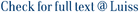The L(2, 1)-labeling of a digraph D is a function l from the vertex set of D to the set of all nonnegative integers such that vertical bar l(x) - l(y)vertical bar &gt;= 2 if x and y are at distance 1, and l(x) not equal l(y) if x and y are at distance 2, where the distance from vertex x to vertex y is the length of a shortest dipath from x to y. The minimum over all the L(2, 1)-labelings of D of the maximum used label is denoted (lambda) over right arrow (D). If C is a class of digraphs, the maximum (lambda) over right arrow (D), over all D is an element of C is denoted (lambda) over right arrow (C). In this paper we study the L(2, 1)-labeling problem on oriented planar graphs providing some upper bounds on (lambda) over right arrow. Then we focus on some specific subclasses of oriented planar graphs, improving the previous general bounds. Namely, for oriented prisms we compute the exact value of (lambda) over right arrow, while for oriented Halin graphs and cacti we provide very close upper and lower bounds for (lambda) over right arrow. (c) 2012 Elsevier B.V. All rights reserved.

L(2,1)-labeling of oriented planar graphs / Calamoneri, Tiziana; Sinaimeri, Blerina. - In: DISCRETE APPLIED MATHEMATICS. - ISSN 0166-218X. - 161:(2013), pp. 1719-1725. [10.1016/j.dam.2012.07.009]

### L(2,1)-labeling of oriented planar graphs

#### Abstract

The L(2, 1)-labeling of a digraph D is a function l from the vertex set of D to the set of all nonnegative integers such that vertical bar l(x) - l(y)vertical bar >= 2 if x and y are at distance 1, and l(x) not equal l(y) if x and y are at distance 2, where the distance from vertex x to vertex y is the length of a shortest dipath from x to y. The minimum over all the L(2, 1)-labelings of D of the maximum used label is denoted (lambda) over right arrow (D). If C is a class of digraphs, the maximum (lambda) over right arrow (D), over all D is an element of C is denoted (lambda) over right arrow (C). In this paper we study the L(2, 1)-labeling problem on oriented planar graphs providing some upper bounds on (lambda) over right arrow. Then we focus on some specific subclasses of oriented planar graphs, improving the previous general bounds. Namely, for oriented prisms we compute the exact value of (lambda) over right arrow, while for oriented Halin graphs and cacti we provide very close upper and lower bounds for (lambda) over right arrow. (c) 2012 Elsevier B.V. All rights reserved.
##### Scheda breve Scheda completa Scheda completa (DC)2013
cacti; halin graphs; l(h; k)-labeling; oriented graphs; prisms
L(2,1)-labeling of oriented planar graphs / Calamoneri, Tiziana; Sinaimeri, Blerina. - In: DISCRETE APPLIED MATHEMATICS. - ISSN 0166-218X. - 161:(2013), pp. 1719-1725. [10.1016/j.dam.2012.07.009]
File in questo prodotto:
File
1-s2.0-S0166218X12002740-main.pdf

Open Access

Tipologia: Versione dell'editore
Licenza: DRM (Digital rights management) non definiti
Dimensione 332.01 kB
Utilizza questo identificativo per citare o creare un link a questo documento: `https://hdl.handle.net/11385/202533`
•6
•7